### 7. MAXIMUM-LIKELIHOOD ERRORS, M-PARAMETERS CORRELATED ERRORS

When M parameters are to be determined from a single experiment containing N events, the error formulas of the preceding section are applicable only in the rare case in which the errors are uncorrelated.. Errors are uncorrelated only for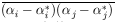= 0 for all cases with ij. For the general case we Taylor-expand w() about (*):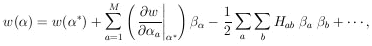whereand(9)

The second term of the expansion vanishes because ðw / ð= 0 are the equations for*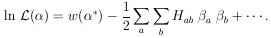Neglecting the higher-order terms, we have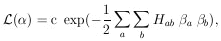(an M-dimensional Gaussian surface). As before, our error formulas depend on the approximation that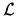() is Gaussian-like in the regionii*. As mentioned in Section 4, if the statistics are so poor that this is a poor approximation, then one should merely present a plot of(). (see Appendix IV).

According to Eq. (9), H is a symmetric matrix. Let U be the unitary matrix that diagonalizes H: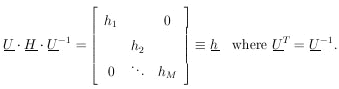(10)

Let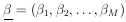and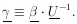. The element of probability in the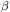-space is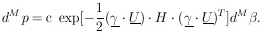Since |U| = 1 is the Jacobian relating the volume elements dMand dM, we have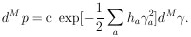Now that the general M-dimensional Gaussian surface has been put in the form of the product of independent one-dimensional Gaussians we have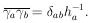Then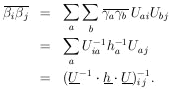According to Eq. (10), H = U-1 . h . U, so that the final result is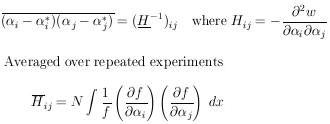Maximum Likelihood Errors, M parameters   (11)

(A rule for calculating the inverse matrix H-1 is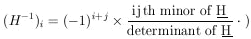If we use the alternate notation V for the error matrix H-1, then whenever H appears, it must be replaced with V-1; i.e., the likelihood function is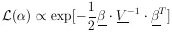(11a)

Example 2

Assume that the ranges of monoenergetic particles are Gaussian-distributed with mean range1 and straggling coefficient2 (the standard deviation). N particles having ranges x1,..., xN are observed. Find1*,2*, and their errors . Then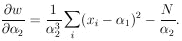The maximum-likelihood solution is obtained by setting the above two equations equal to zero.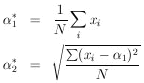The reader may remember a standard-deviation formula in which N is replaced by (N - 1):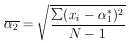This is because in this case the most probable value,2*, and the mean,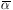2 , do not occur at the same place. Mean values of such quantities are studied in Section 16. The matrix H is obtained by evaluating the following quantities at1* and2*: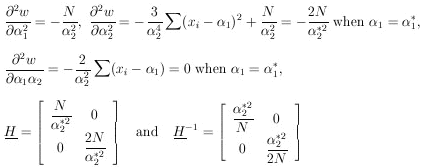According to Eq. (11), the errors on1 and2 are the square roots of the diagonal elements of the error matrix, H-1: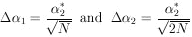(this is sometimes called the error of the error).

We note that the error of the mean is 1/sqrt[N]where=2 is the standard deviation. The error on the determination ofis/sqrt[2N].

Correlated Errors

The matrix Vijis defined as the error matrix (also called the covariance matrix of). In Eq. 11 we have shown that V = H-1 where Hij = - ð2 w / (ði ðj). The diagonal elements of V are the variances of the's. If all the off-diagonal elements are zero, the errors inare uncorrelated as in Example 2. In this case contours of constant w plotted in (1,2) space would be ellipses as shown in Fig. 2a. The errors in1 and2 would be the semi-major axes of the contour ellipse where w has dropped by ½ unit from its maximum-likelihood value. Only in the case of uncorrelated errors is the rms errorj = (Hjj) and then there is no need to perform a matrix inversion.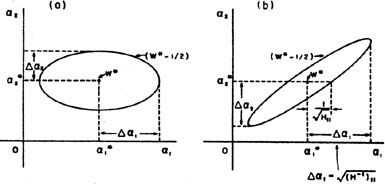Figure 2. Contours of constant w as a function of1 and2. Maximum likelihood solution is at w = w*. Errors in1 and2 are obtained from ellipse where w = (w* - ½). (a) Uncorrelated errors. (b) Correlated errors. In either case12 = V11 = (H-1)11 and22 = V22 = (H-1)22. Note that it would be a serious mistake to use the ellipse "halfwidth" rather than the extremum for.

In the more common situation there will be one or more off-diagonal elements to H and the errors are correlated (V has off-diagonal elements). In this case (Fig. 2b) the contour ellipses are inclined to the1,2 axes. The rms spread of1 is still1 = sqrt[V11], but it is the extreme limit of the ellipse projected on the1-axis. (The ellipse "halfwidth" axis is (H11) which is smaller.) In cases where Eq. 11 cannot be evaluated analytically, the*'s can be found numerically and the errors incan be found by Plotting the ellipsoid where w is 1/2 unit less than w * . The extremums of this ellipsoid are the rms error in the's. One should allow all thej to change freely and search for the maximum change ini which makes w = (w * - ½). This maximum change ini, is the error ini and is sqrt[V11].